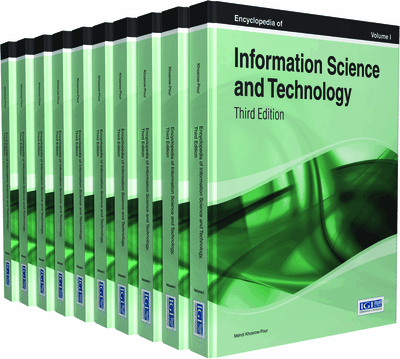# Image Segmentation Methods

Manassés Ribeiro, Heitor Silvério Lopes
DOI: 10.4018/978-1-4666-5888-2.ch588
OnDemand:
(Individual Chapters)
Available
\$29.50
No Current Special Offers

Top

## Background

### Image Segmentation

In bitmap graphics (not in vector graphics) segmentation is a pre-processing step in computer vision where images are partitioned into several distinct regions formed by a set of pixels (picture element). Such regions are further labeled as foreground (objects of interest) or background, based on the common properties the pixels of a region must share (such as color, intensity, texture, etc).

Several image segmentation methods were proposed in the literature. However, a single method may not be efficient for a specific image class, and a combination of them is necessary to solve interesting real-world problems. The main methods for image segmentation are based on histogram analysis, edge detection and segmentation by regions.

### Histogram Analysis

A histogram is a graphical representation in which a data set is grouped into uniform classes such that the horizontal axis represents the classes and, the vertical axis, the frequencies in which the values ​​of these classes are present in the data set. Based on the central tendency or histogram variation it is possible to determine the cutoff point that will be used as threshold in these segmentation process. In this approach classes with high and low frequency are identified where a class with low frequency between two high frequency classes usually represents the best cutoff point to image threshold. An example of histogram analysis is presented in Figure 1 (b) where classes with high and low frequencies can be seen.

An efficient approach for image segmentation based on histogram analysis is the Otsu method (Otsu, 1979). This method performs several iterations analyzing all possible thresholds to look for the best threshold T that presents the highest inter-class variance. This method assumes that the image to be segmented will be classified in two classes, object and background, and threshold point will be determined by the pixel intensity value that represents the minimum intra-class variance. This threshold is exhaustively searched and can be defined as a weighted sum of the variances of the two classes, as shown in Equation 1:

(1) where σ represents the variance of these classes and weights Wi represents the occurrence probability of each class being separated by a threshold T. Figure 1 (a) shows the original color image and Figure 1 (c) shows image segmented by Otsu approach.

## Key Terms in this Chapter

Gradient: A gradual transition between one or more colors. In mathematics, the gradient is a generalization of the usual concept of derivative to functions of several variables.

Genetic Programming: It is a specialization of genetic algorithms where each individual is a computer program and consists in is an evolutionary algorithm-based methodology to find computer programs.

Computer Vision: A discipline of Computer Science that includes methods for acquiring, processing, analyzing, and understanding.

Genetic Algorithm: Belongs to the larger class of evolutionary algorithms and is a search heuristic inspired on process of natural selection and is routinely used to generate useful solutions to optimization and search problems.

Region Growing: Simple region-based image segmentation method. It is also classified as a pixel-based image segmentation method since it involves the selection of initial seed points.

Convolution Masks: In image processing convolution mask is a small matrix with a set of weightings which is applied to pixel values in order to create a new effect such as blurring, sharpening, embossing, edge-detection, and more.

Pixel: Short for picture element, it is physical point in a bitmap image, or a smaller addressable element in an all points of an addressable display device.

Image Segmentation: Process of partitioning a digital image into object(s) and background. The goal of segmentation is to simplify the representation of an image into something that is more meaningful and easier to analyze.

Evolutionary Computation: Subfield of computational intelligence that involves continuous optimization and combinatorial optimization problems and can be considered global optimization methods.

Edge Detector: It is mathematical methods which aim at identifying points where the image brightness changes sharply, or discontinuities, in a digital image.

## Complete Chapter List

Search this Book:
Reset